Why don't we directly solve for the field in FEM?

Today, we’ll be talking about FEM a bit. FEM, aka the finite element method, is a numerical method for finding approximate solutions for partial differential equations. However, we usually obtain the solution via a potential of some kind, rather than directly solving for the quantity of interest. For me, that’s usually the vector potential.

But why do we take this convoluted route? This post will answer that question. Since this such a mathematical and equation-heavy topic, here’s a picture of a cat:The cat wants to know why the vector potential is so commonly used. Can you explain, or are you going to disappoint them?

### What to solve?

In my line of work, FEM is typically used to solve the Maxwell’s equations. In other words, the fundamental equations describing the dependence between magnetic and electric fields and flux densities. Since the frequencies included are usually somewhat low (compared to radio signals), we typically exclude the so-called displacement current term. This approximation is called quasi-static.

Let’s use the simplest case as an example, i.e. no eddy currents. Then, we only need three equations:

(1)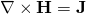(2)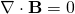(3)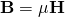The first of these basically tells us how the magnetic field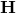circles around any currents in the problem domain. The second one, with the divergence operator, says that no magnetic flux density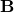is created out of nothing. No magnetic monopoles so far, that is. Sorry. Finally, the last equation tiesandtogether through the material-dependent magnetic permeability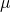.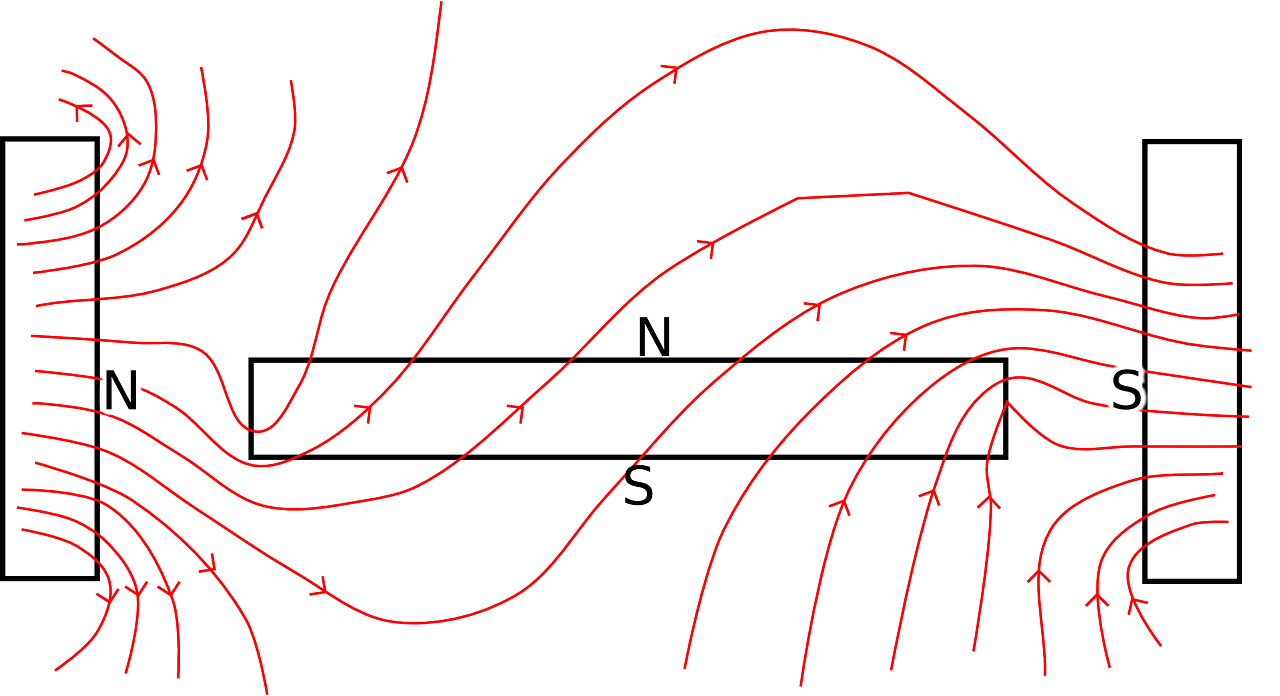A typical field problem looks a bit like this.

### How we solve it

So how do we solve this problem?

Well, we typically begin by determining a new quantity, the so-called vector potential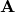. Specifically, we want the following relationship to hold betweenand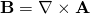.

Nicely for us, this definition satisfies equation (2) automatically. Because of this, we can simply substitute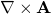into (3) and (1), to get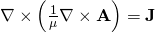.

As you can see, we managed to reduce three equations into only one. Quite nice, isn’t it?

Anyway, this final equation we can then solve with our FEM tricks, and a bit of computing power.

#### Not so fast

Technically, the above is fully accurate only in 2 dimensions. You see, the relationdoes not define a unique. Indeed, if we add any gradient field to, the resulting flux density will still be the same. Like this: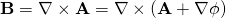for any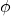.

To ascertain the uniqueness, the so-called Coulomb’s gauge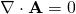.

is commonly used.

In typical 2-dimensional magnetic problems, this is satisfied automatically for the following reason. In order forto lie in the xy-plane,has to be purely z-directional. Since no z-dependency is assumed, it’s divergence will obviously be zero.

But, in 3D analysis the gauge will be taken into account by other means.

### Why the potential?

Okay, so the vector potential works very nicely in 2D. But why is it still used in 3D problems? You might think that it might be just as easy to solve the Maxwell’s equations directly from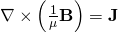rather than going through the gauged vector potential formulation.

To answer this, we need to briefly consider how the finite element method works.

#### They just work quite nicely

Basically, the idea is to writewith a weighted sum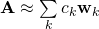with some known functions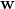and unknown coefficients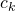.

However, the nature of the underlying physical problem sets some requirements for the functions. Using a little bit of maths, it is possible to show that the tangential component of the vector potential has to be continuous over any possible boundary in the problem. Sinceis a linear combination of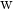, this is easily satisfied if the tangential components ofare continuous.

The so-called edge elements fulfil this requirement, while at the same time fulfilling the gauge condition. Talk about a win-win. Thus, they are very commonly used in 3D analysis.

Well to be honest, edge elements do not fulfil the  gauge condition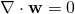automatically. It either has to be enforced manually, or dealt with using other means. But we take what we can get.

This same tangentiality requirement for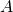andcan also be deduced from the potential formulation itself.  By using the Galerkin approach and doing a simple integration by parts, the left side of the curl-curl equation is reduced to a matrix with entries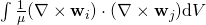.

If our functionsare tangentially continuous, the curls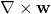will be finite everywhere. Hence, the integral is easy to evaluate.

#### Life without potentials

Let’s see what would happen if we tried to solve fordirectly. One of the easiest-to-understand ways would be to minimize the squared error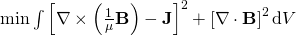with the Galerkin’s method. Indeed, this is what the least-squares finite element method is about.

However, this approach would result in terms like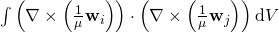and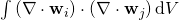in our discretized equation.

However, now we see (you do see, right? RIGHT??) that

a) The functions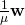should to be tangentially continuous, lest the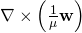term blow up

and

b) The functionsshould to be normally continuous, or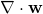blows up.

In case there are abrupt changes in(like, say, in an electrical machine) these two requirements are quite damn hard to satisfy at the same time.

Indeed, some special tricks would probably have to be applied, like trying to minimise the norm of the said discontinuities in the functions. However, these penalty methods are known to suffer from convergence issues, especially in nonlinear problems.

Indeed, the least squares methods, in general, seem pretty much unsuitable for the analysis of electrical machines. There are some esoteric adjoint-norm-based mathematical nightmare-approaches, but they don’t work well in any realistic problems.

I tried. Don’t do the same mistake.

Still awake? Here, have a cookie.

-Antti

Need help with electric motor design or design software? Let's get in touch - satisfaction guaranteed!Why Bother with the Vector Potential

### 2 thoughts on “Why Bother with the Vector Potential”

•Manoj says:

thanks for giving the basics..I couldn’t understand the following part. Would you please elaborate?
” the tangential component of “H” is continuous over any boundary within our problem. In case the permeability \mu has a jump on the said boundary, the tangential component of “A” has to be discontinuous.

On the other hand, in the optimal case “w” should also satisfy the gauge condition Div(“w”) = 0. Of course, this is a slight contradiction with the normal-discontinuity requirement above – having a normal jump of course results in a singularity in the divergence. However, the gauge condition is still satisfied almost everywhere, which is enough to guarantee uniquity”

1.Antti (admin) says:

Thank you for the very good question! It got me thinking, and I realized I had confused a little bit something. Indeed, the tangential continuity is primarily what is needed with the vector potential formulation. Technically, A should also be normally continuous to satisfy the gauge condition everywhere, but that requirement is usually relaxed. So normal discontinuity is definitely not NEEDED, but simply tolerated.

Now, the possibility for a normal discontinuity IS a characteristic of the edge shape functions. But, that is mainly useful when solving full-wave equations by representing H directly with edge elements (since normal component of H has a jump whenever the permeability has).

I’ve now revised the post a bit 🙂